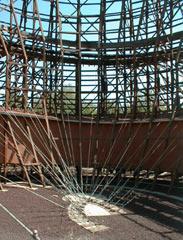Ch 2. Vectors Multimedia Engineering Statics Scalars & Vectors 2-DVectors 3-DVectors DotProducts
 Chapter 1. Basics 2. Vectors 3. Forces 4. Moments 5. Rigid Bodies 6. Structures 7. Centroids/Inertia 8. Internal Loads 9. Friction 10. Work & Energy Appendix Basic Math Units Sections eBooks Dynamics Fluids Math Mechanics Statics Thermodynamics Author(s): Kurt Gramoll ©Kurt GramollChapter 2 VectorsTopics Reviewed Scalars, Vectors, and Operations 2-D Vectors 3-D Vectors Dot Products The topic menu above allows you to move directly to any of the four sections for each topic. The sections are: Case Intro: To help introduce and understand the basic principles, a case study is presented. Theory: This section will review the basic principles and equations that you should know to answer the exam questions. It does not give detailed derivations of the theory. Case Solution: The case study is solved in detail in this section. Graphics, narrations, animations, and equations are used to help you understand how the problem was solved. Example: Addition example problem(s) for some sections.